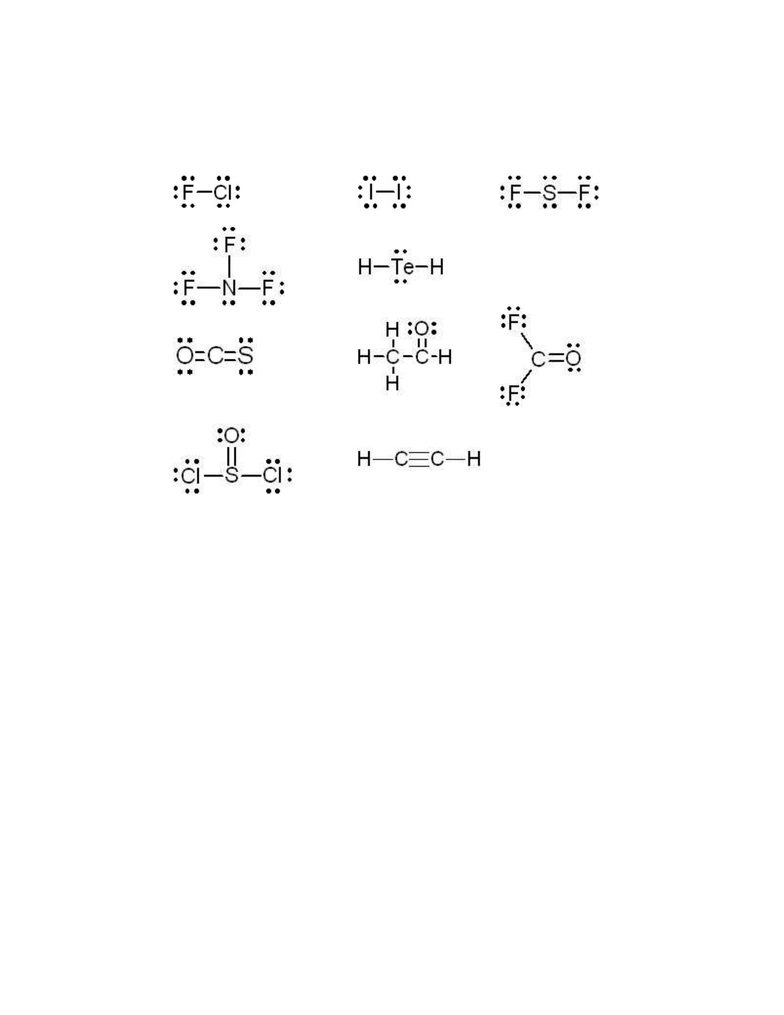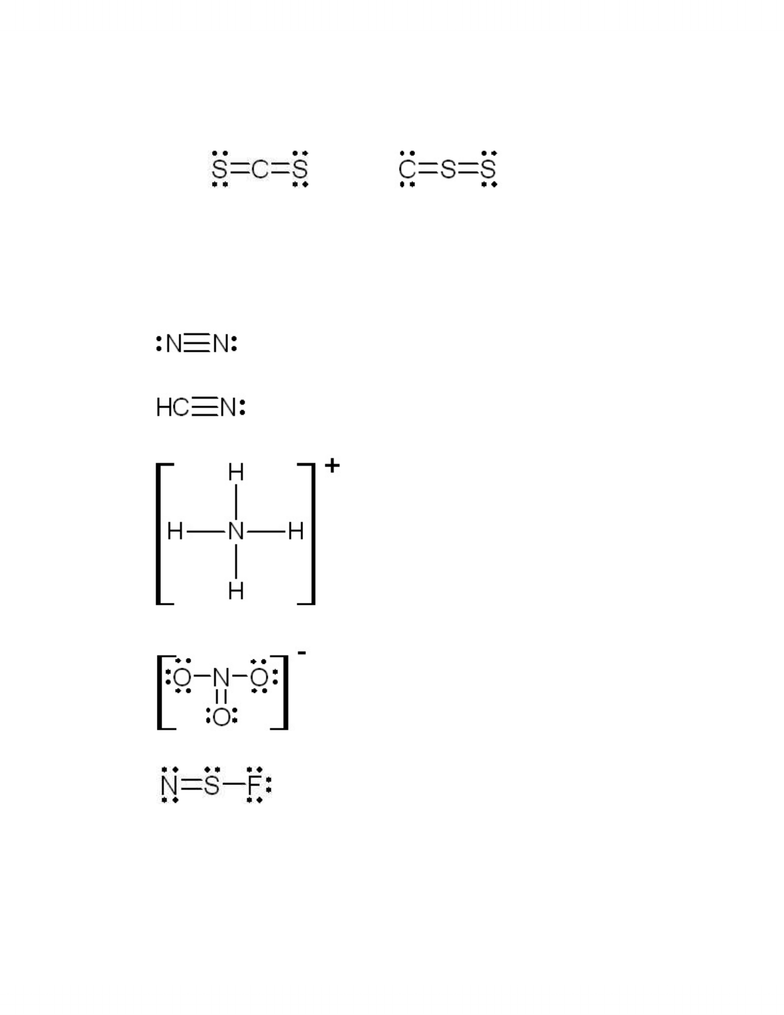# bonding notes

121 views7 pages
School
Department
CoursePetrucci 10 ed Problem set #4: Chemical bonding 1 of 7
th
Petrucci 10 Edition problem set #4: Chemical Bonding
th
Chapter 10
10.3a) b) c)
d) e)
10.4a) b) c)
d) e)
10.11 a) incomplete octet around C
b) incomplete octet around left C and not enough valence electrons in the
structure
c) this Lewis structure is correct
d) too many valence electrons in this structure
10.17 a) FC(H) = 1 – 0 – 1 = 0
FC(central C) = 4 – 0 – 4 = 0
FC (terminal C) = 4 – 2 – 3 = -1
b) FC(C) = 4 – 0 – 4 = 0
FC(-O) = 6 – 6 – 1 = -1
FC(=O) = 6 – 4 –2 = 0
c) FC(H) = 1 – 0 – 1 = 0
FC(central C) = 4 – 0 – 3 = +1
FC (terminal C) = 4 – 0 – 4 = 0
10.18 a) FC(I) = 7 - 6 - 1 = 0
b) FC(S) = 6 – 2 - 3 = +1
FC(-O) = 6 – 6 - 1 = -1
FC(=O) = 6 – 4 - 2 = 0
c) FC(N) = 5 – 1 - 3 = +1
FC(-O) = 6 – 6 - 1 = -1
FC(=O) = 6 – 4 - 2 = 0
www.notesolution.com
Unlock document

This preview shows pages 1-2 of the document.
Unlock all 7 pages and 3 million more documents.Petrucci 10 ed Problem set #4: Chemical bonding 2 of 7
th
10.21 a) FC(O) = 6 - 2 - 3 = +1 b) FC(Al) = 3 - 0 - 4 = -1c) FC(Cl) = 7 - 2 - 5 = 0
d) FC(Si) = 4 - 0 - 6 = -2e) FC(CI) = 7 - 4 - 3 = 0
10.22 b)
FC(S) = 6 - 4 - 2 = 0 FC(C) = 4 - 4 - 2 = -2
central
FC(C) = 4 - 0 - 4 = 0 FC(S) = 6 - 0 - 4 = +2
terminal
FC(S) = 6 - 4 - 2 = 0
The structure on the left is the most plausible.
10.59 a) linear
b) linear
c) tetrahedral
d) trigonal planar
e) bent
www.notesolution.com
Unlock document

This preview shows pages 1-2 of the document.
Unlock all 7 pages and 3 million more documents.

## Document Summary

Petrucci 10 edition problem set #4: chemical bonding th. Petrucci 10 ed problem set #4: chemical bonding 1 of 7 th. 10. 11 a) incomplete octet around c: incomplete octet around left c and not enough valence electrons in the structure, this lewis structure is correct, too many valence electrons in this structure. Fc(central c) = 4 0 4 = 0. Fc (terminal c) = 4 2 3 = -1. Fc(central c) = 4 0 3 = +1. Fc (terminal c) = 4 0 4 = 0. Fc(=o) = 6 4 - 2 = 0 www. notesolution. com. Petrucci 10 ed problem set #4: chemical bonding 2 of 7 th. = 6 - 4 - 2 = 0 central terminal. The structure on the left is the most plausible. 10. 59 a) linear linear tetrahedral trigonal planar bent www. notesolution. com. Petrucci 10 ed problem set #4: chemical bonding 3 of 7 th. Petrucci 10 ed problem set #4: chemical bonding 4 of 7 th.

## Get access